﻿ Two-arm probes in atomic force microspopy

# Size of an atom ?

## We have the technology of moving atom !

### [ Atomic force microscope can move tip very small distance. ]

(Fig.1)  We can control and move the tip very small distance ( < 0.1 Å ) !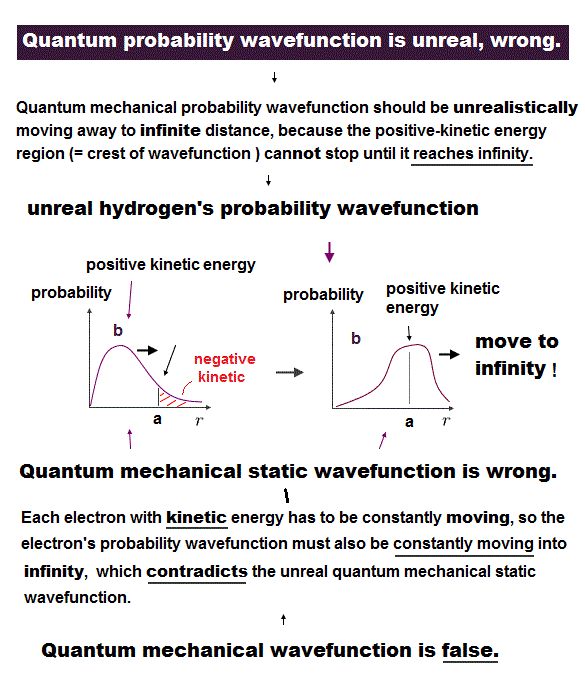In atomic force microscopy, we can observe and manipulate each single atom, using actuator .

It means we already have the technology of moving the tip of microscope very small distance ( < 0.1 Å ) to distinguish a single atom.

But under the useless quantum mechanics, we cannot move forward to the next step of making artificial molecules by controling each atom !

## Two probes is needed to control each atom !

### [ Only a single probe cannot even identify each atom. ]

(Fig.2)  ↓ A single probe cannot feel true "force" specific to each atom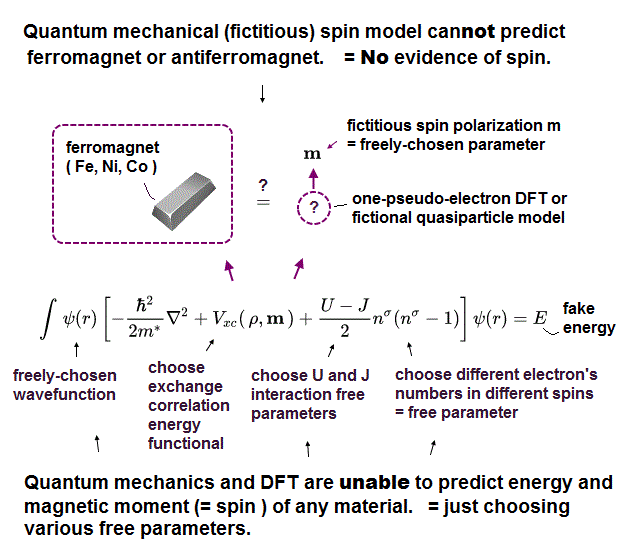All the present atomic force microscopes have only one single probe tip, which can detect only "relative" force to identify each atom.

When you press the tip of probe on some target atom. the force it can detect is not the true force specific to each atom.

The single probe can detect the force2 in Fig.2 between the whole molecule and the ground under it.

To know the true atomic force and radius specific to each atom, we need at least two probes, and put each atom between those probes !

Using two probe tips, not only identifying but also picking up each atom are possible !

## How big is an atom ?

### [ Uselss quantum mechanics doesn't tell us the size of each atom ! ]

(Fig.3)  Carbon and silicon with same valence electrons, but different sizes.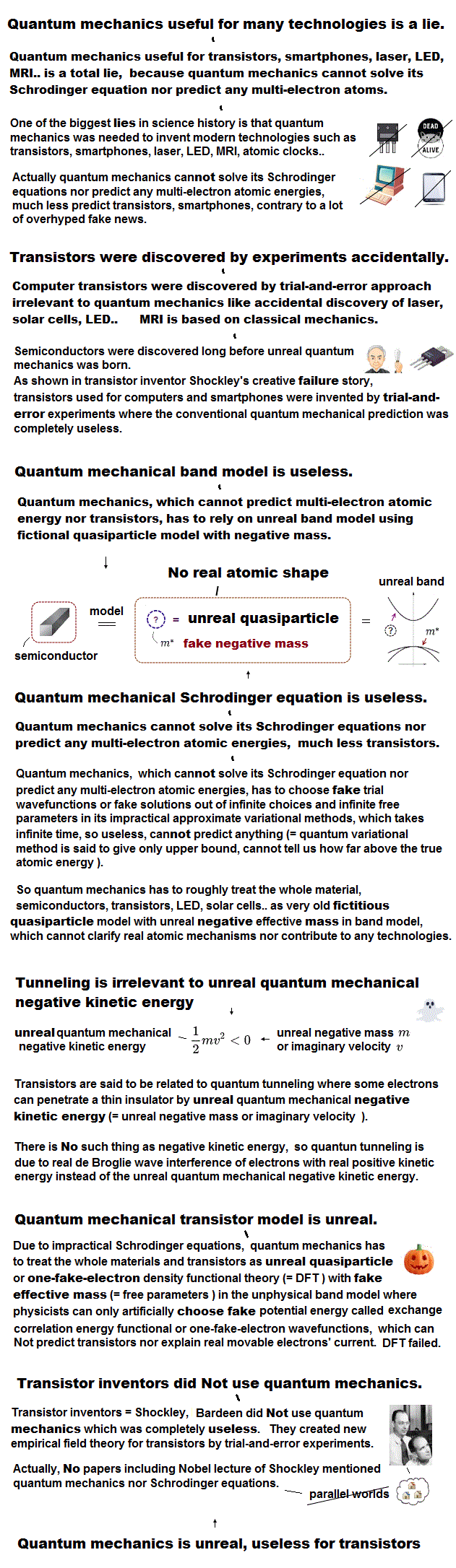It is known that both carbon and silicon atoms have the same four valence electrons, but have the different atomic sizes.

Because the molecular bond lengths of carbon and silicon are always different.  Quantum mechanics cannot tell us the clear size of each atom !

Because quantum mechanics wave function has NO realistic concepts such as "separated electrons".

## What determines the size of each atom ?

### [ Some real object besides Coulomb force is needed to determine the atomic size. ]

(Fig.4)  Bond lengths between C-H and Si-H are different.  Why ?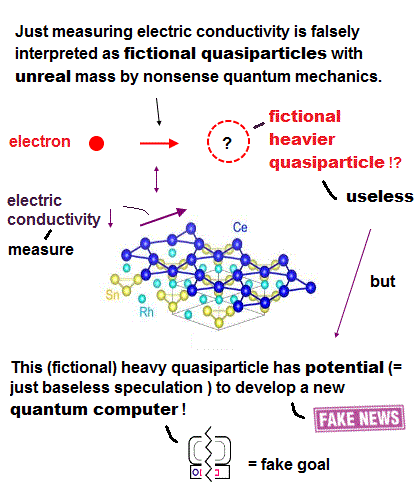Both cabon and silicon have the same valence electrons and similar properties, but their molecular bond lengths are different.

Silicon (= Si ) bond length is always longer than the carbon (= C ).  What determines these bond lengths specific to each atom ?

Unfortunately, useless quantum mechanics cannot show clear reason for these atomic size and bond length.

Because quantum mechanics does NOT allow us to introduce any real objects such as "separated electrons".  It only shows vague wave function.

## Real de Broglie wave is necessary.

### [ Quantum mechanics doesn't admit real de Broglie wave ! ]

(Fig.5)  As de Broglie wavelength increases, the atom becomes bigger.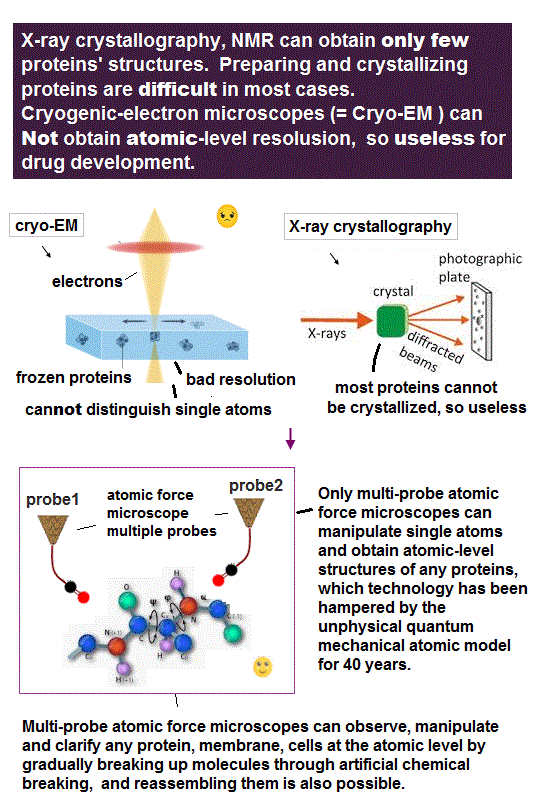Many experiments proved that an electron is actually de Broglie wave, and causes inteference in double-slit.

Despite those proofs, quantum mechanics never admit the existence of real de Broglie wave, and it gives up reality !

It is known that as de Broglie wavelength increases, the atomic radius increases.  Carbon ( or silicon ) orbit is 2 ( or 3 ) × de Broglie wavelength.

From the ionization energies and this de Broglie wavelength, we can estimate each atomic radius, which agrees with experimental bond lengths.

In non-covalent bond, we have to consider Pauli exclusion effect after occupying de Broglie wave's holes.

## "Real" atomic model is necessary.

### [  ① Introduce real atomic model → ② formulate atomic force  ]

(Fig.6)  Introducing real electrons' motion, real de Broglie wave is essential.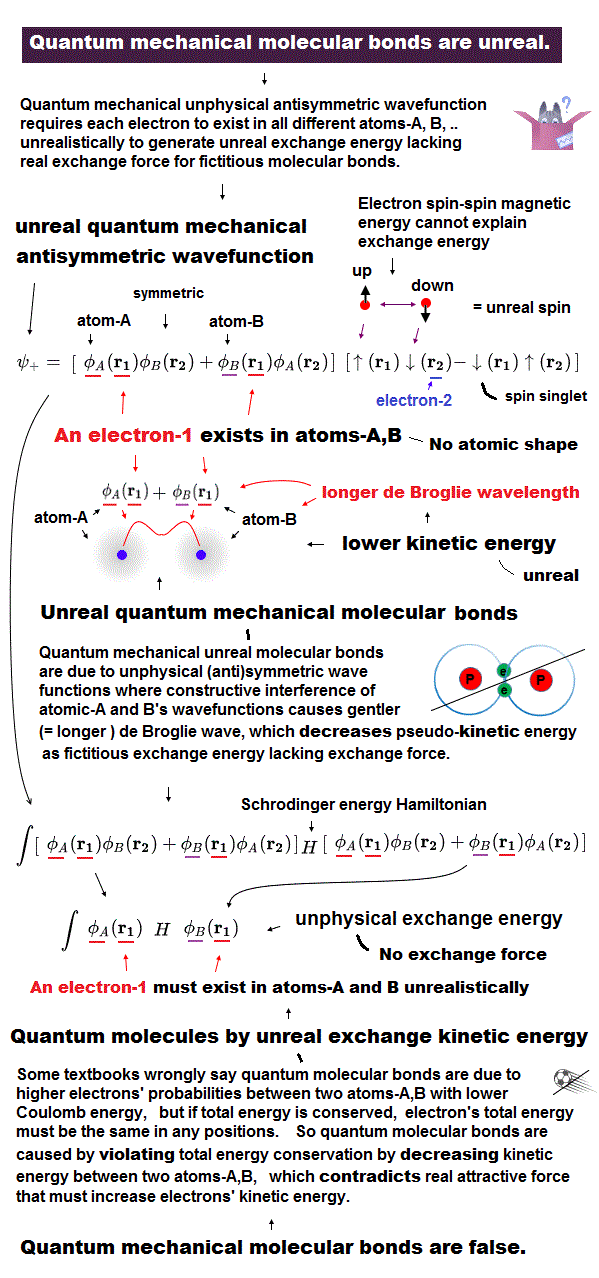We can measure each atomic force using atomic force microscope, but quantum mechanics cannot formulate these forces using 'concrete' models !

The first thing to do is introduce real and simple atomic models.  Quantum useless wave function cannot separate each single electron.

So in Schrödinger equation, two electrons cannot avoid each other by Coulomb repulsions in the simple way, which makes it very hard to formulate atomic force.

Not only Coulomb forces but also de Broglie wave is necessary to estimate each atomic radius ( atomic radius is longer as 1, 2, 3 × .. de Broglie wavelength ).

Interference among electrons' de Broglie waves can explain well about Pauli exclusion force and valence electron number.

Quantum Schrödinger equation does NOT admit real de Broglie wave, so it cannot estimate actual atomic radius.

So under vague quantum wave function (= fake solution in multi-electrons ), it's impossible to formulate actual interatomic force and atomic tweezers.

## Useless quantum mechanics is the only option !

### [ Quantum mechanics has NO solution in multi-electron atoms. ]

(Fig.7)  They just choose "fake solution" from infinite choices ← useless !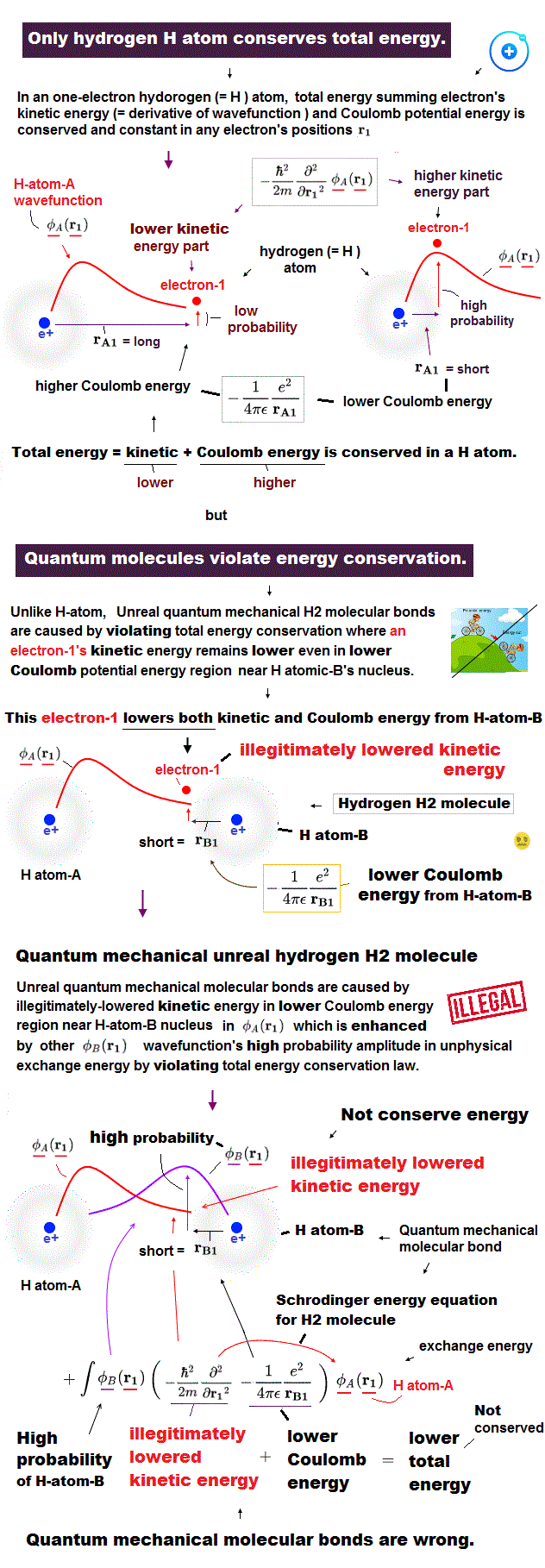After detecting the slight force of a single atoms or molecule, we need to interpret those forces using some atomic model.

Unless we can explain each single atomic force, we cannot move forward to the next step of utilizing multi-atomic bahavior.

The problem is that there is the only useless quantum mechnaics (= Schrödinger equation ) as a tool to interpret these atomic data, now.

Quantum mechanics have NO exact solution in multi-electron atoms, so they just choose fake solution from infinite choices, meaning no power to predict anyhing.

In atomic force microspopy, they use densisty functional theory (= DFT ), which is a further approximation of quantum mechanics, so more incorrect and useless.

## Density functional theory (= DFT ) is useless.

### [ DFT just "chooses" fake potential from infinite choices, so useless. ]

(Fig.8)  DFT is one of "false" approximations of Schrödinger equation.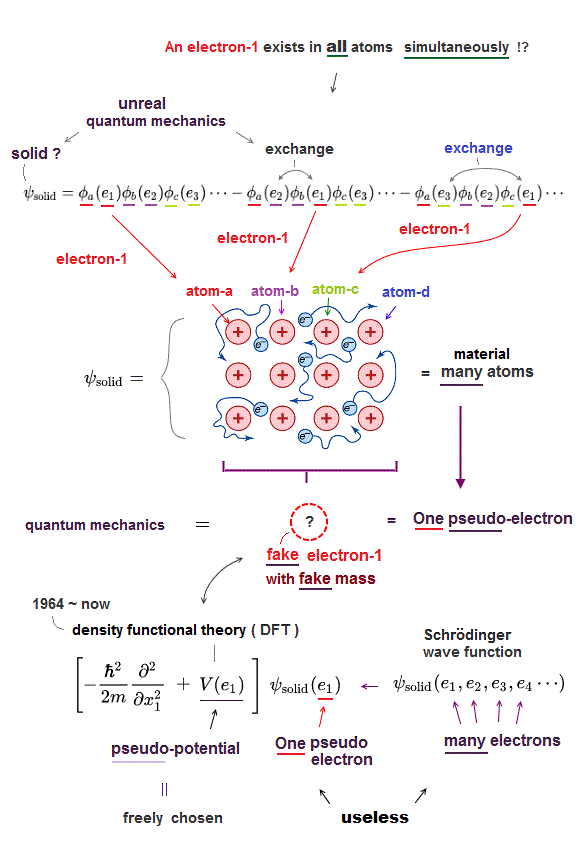In atomic force microcopy, the only tool is useless density functional theory (= DFT ).  All papers rely on this DFT ( this , this ).

Density functional theory (= DFT ) is one of approximate Schrödinger equations.  DFT replaces electron-electron interaction by fake artificial potential.

In Schrödinger equation, they just choose fake approximate solutions as "trial wavefunction".  In DFT, they have to choose "fake artificial potential", too.

There are NO restrictions in choosing these fake potentials.  We can choose any forms of these potentials from infinite choices in DFT.

So DFT has NO ability to predict any atomic behavior.  In spite of this, this DFT is the only tool for analysing atomic force microcsopy !

No matter how many times they catch slight atomic force, all they can use is useless density functional theory (= DFT, see this p.6 ).

All in these single molecule detections, useless density functional theory is the only method to interpret each atomic force ( see abstract of this and this ).

This is where out science stops.  Physicists are shackled to old useless methods which don't admit "concrete force".  It just gives us weird wavefunctions as a tool.

## Choosing "fake potential" in DFT.

### [ DFT chooses fake potential, solution from infinite choices. ]

(Fig.9)  So DFT cannot predict any atomic behavior, useless.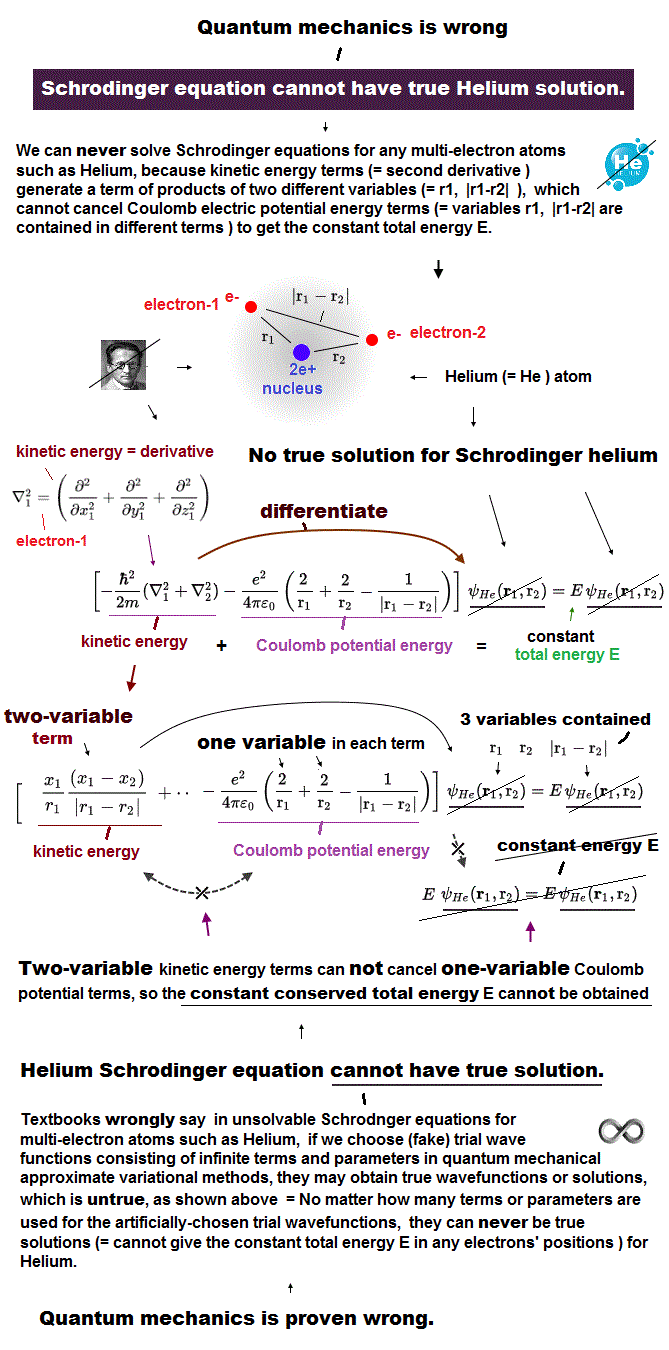Density functional theory (= DFT ) forcedly changes muti-electron equation into one-electron approximation.

In one-electron DFT method, we need to artificially pick up "fake" forms of electrons' interaction potential called exchange correlation functional.

Selecting convenient functional for your purpose is all you have to do in DFT ( this p.9 ).  There is NO universal functional describing any potential ( this p.2 ).

When you pick up one of functional ( ex. LDA ) from infinite choices and it fails, you can pick up other functionals as fake electrons' potential.

Selecting fake potential and fake solutions as you like means this DFT method has NO ability to predict any atomic behavior, so useless, too2017/3/31 updated. Feel free to link to this site.